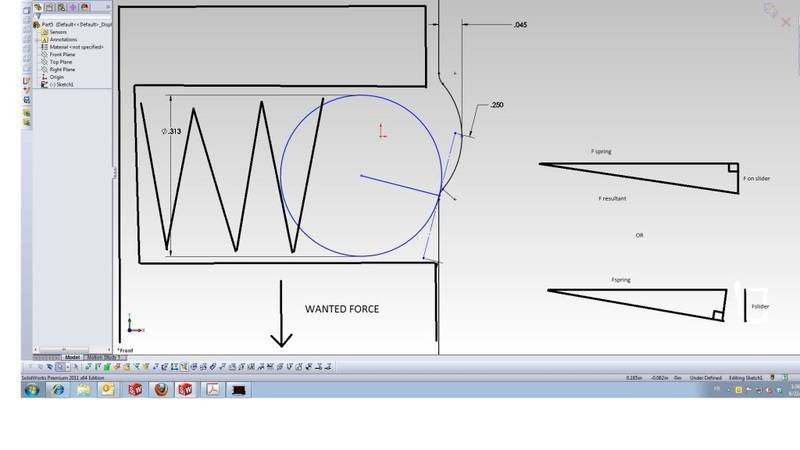# Spring Force Resultant help please

gogogsr
Hey guys, how are you?

I feel so stupid right now, I'm having a hard time getting my mind straight, which of the two resultant force diagram on the right is right? Typically its a slider with a ball and spring that slides on a recess (cam type). I want to know the force required on the slider to make it slide, here is the diagram. Thanks guysHomework Helper
hi gogogsr!the diagram on the left clearly shows the applied force as being in the -y direction(and btw, if there isn't another surface on the left (or some unshown x direction force), how does the slider stay in contact with the surface on the right?)

gogogsr
there is a surface on the left, it is just a quick diagram. The question is: Is the Fspring the resultant force (hypotenuse) of the triangle or just the x vector. I'm thinking it should be the resultant since it is the only applied external force , so it as to be the greatest force. (I know there is friction and everything, but keep it simple please)

Studiot
Is this a continuation of this thread?

To keep it simple the spring force pushes the ball against the detent with force F given by the spring constant times the extension.

This spring force is equal to the normal contact force so the force perpendicular to this is your 'wanted force'. This in turn is the friction force which is the normal force times the coefficient of friction.

Last edited:
gogogsr
could you draw it please ;-)

Homework Helper
hi gogogsr!I'm thinking it should be the resultant since it is the only applied external force , so it [h]as to be the greatest force.

i'm sorry, but this is nonsense, if you think like this it'll only confuse youthere are only two ways you can find the spring force …

i] from the spring displacement, using hooke's law (though you may not know the displacement, or the spring constant accurately enough for that)

ii] by choosing the whole or part of the system, drawing a free body diagram for it, and summing all the forces

(i still don't understand exactly what you're trying to investigate, but if it's the difference in spring force between the two extreme positions, then that will be simply the depth of the recess times the spring constant)